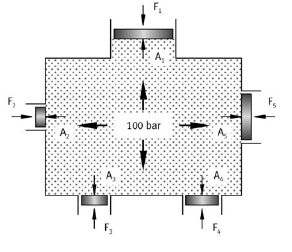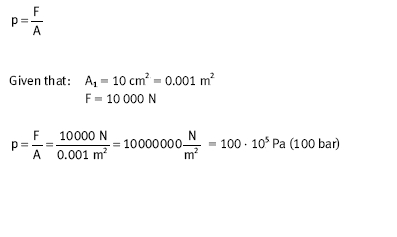﻿ Pressure transmission – Hydraulic Schematic Troubleshooting

## Pressure transmission

If a force FT1T acts via an area AT1T on an enclosed liquid, a pressure p is produced which extends throughout the whole of the liquid (Pascal’s Law). The same pressure applies at every point of the closed system (see diagram).Pressure transmission

Owing to the fact that hydraulic systems operate at very high pressures, it is possible to neglect the hydrostatic pressure (see example). Thus, when calculating the pressure in liquids, the calculations are based purely on pressure caused by external forces. Thus, the same pressure acts on the surfaces AT2T, AT3T as on AT1T. For solid bodies, this is expressed by means of the following formula: